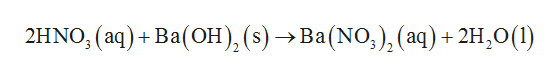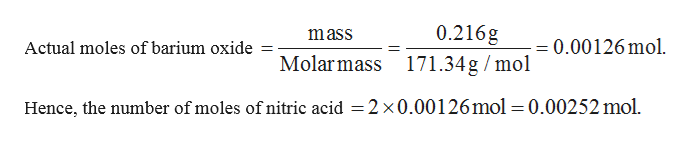What is the molarity of a solution of nitric acid if 0.216 g of barium hydroxide is required to neutralize 20.00 mL of nitric acid?

Question
• What is the molarity of a solution of nitric acid if 0.216 g of barium hydroxide is required to neutralize 20.00 mL of nitric acid?
Step 1

The balanced neutralization reaction between barium hydroxide and nitric acid is given below.help_outlineImage Transcriptionclose2HNO, (aq) + Ba(Oн), (s) > Ва(NO,), (аq) + 2H,0(1) fullscreen
Step 2

Molar mass of barium hydroxide is 171.34 g/mol. From the above equation, it can be stated that for neut...help_outlineImage Transcriptionclose0.216g Molarmass 171.34g/mol mass 0.00126 mol Actual moles of barium oxide Hence, the number of moles of nitric acid = 2 x0.00126mol=0.00252 mol fullscreen

Want to see the full answer?

See Solution

Want to see this answer and more?

Our solutions are written by experts, many with advanced degrees, and available 24/7

See Solution
Tagged in

Quantitative analysis